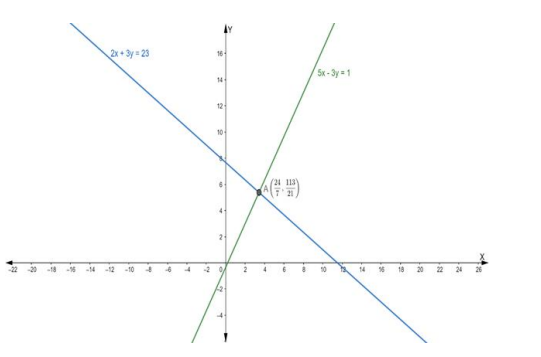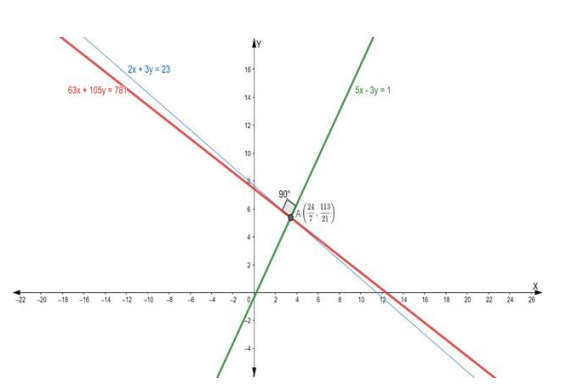# Find the equation of the line through the intersection of the lines`
Question:

Find the equation of the line through the intersection of the lines $5 x-3 y=1$ and $2 x+3 y=23$ and which is perpendicular to the line $5 x-3 y=1$

Solution:

Suppose the given two lines intersect at a point $P\left(x_{1}, y_{1}\right) .$ Then, $\left(x_{1}, y_{1}\right)$ satisfies each of the given equations.

$5 x-3 y=1 \ldots(i)$

$2 x+3 y=23 \ldots$ (ii)

Now, we find the point of intersection of eq. (i) and (ii)

Adding eq. (i) and (ii) we get

$5 x-3 y+2 x+3 y=1+23$

$\Rightarrow 7 x=24$

$\Rightarrow x=\frac{24}{7}$

Putting the value of x in eq. (i), we get

$5\left(\frac{24}{7}\right)-3 y=1$

$\Rightarrow \frac{120}{7}-3 \mathrm{y}=1$

$\Rightarrow-3 \mathrm{y}=1-\frac{120}{7}$

$\Rightarrow-3 \mathrm{y}=\frac{7-120}{7}$

$\Rightarrow-3 \mathrm{y}=-\frac{113}{7}$

$\Rightarrow \mathrm{y}=\frac{113}{21}$

Hence, the point of intersection $\mathrm{P}\left(\mathrm{x}_{1}, \mathrm{y}_{1}\right)$ is

$\left(\frac{24}{7}, \frac{113}{21}\right)$Now, we know that, when two lines are perpendicular, then the product of their slope is equal to $-1$

$m_{1} \times m_{2}=-1$

$\Rightarrow$ Slope of the given line $\times$ Slope of the perpendicular line $=-1$

$\because \frac{5}{3} \times$ Slope of the perpendicular line $=-1$

$\Rightarrow$ The slope of the perpendicular line $=-\frac{3}{5}$

So, the slope of a line which is perpendicular to the given line is $-\frac{3}{5}$

Then the equation of the line passing through the point $\left(\frac{24}{7}, \frac{113}{21}\right)$ having slope

$-\frac{3}{5}$ is:

$y-y_{1}=m\left(x-x_{1}\right)$

$\Rightarrow \mathrm{y}-\left(\frac{113}{21}\right)=-\frac{3}{5}\left(\mathrm{x}-\frac{24}{7}\right)$

$\Rightarrow 5 \mathrm{y}-5 \times \frac{113}{21}=-3 \mathrm{x}+\frac{24}{7}$

$\Rightarrow 5 \mathrm{y}-\frac{565}{21}=-3 \mathrm{x}+\frac{72}{7}$

$\Rightarrow 3 \mathrm{x}+5 \mathrm{y}-\frac{565}{21}-\frac{72}{7}=0$

$\Rightarrow \frac{63 \mathrm{x}+105 \mathrm{y}-565-216}{21}=0$

$\Rightarrow 63 x+105 y-781=0$